The magnetic susceptibility is negative for

1. paramagnetic material only

2. ferromagnetic material only

3. paramagnetic and ferromagnetic materials

4. diamagnetic material only

Concept Questions :-

Magnetic materials
High Yielding Test Series + Question Bank - NEET 2020

Difficulty Level:

The following figures show the arrangement of bar magnet in different configurations. Each magnet has magnetic dipole moment m. Which configuration has the highest net magnetic dipole moment?
(i)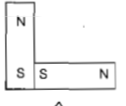(ii)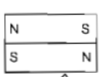(iii)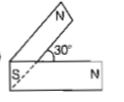(iv)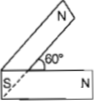1. (i)

2. (ii)

3. (iii)

4. (iv)

Concept Questions :-

Bar magnet
High Yielding Test Series + Question Bank - NEET 2020

Difficulty Level:

A compass needle which is allowed to move in a horizontal plane is taken to a geomagnetic pole.It

(a) will become rigid showing no movement

(b) will stay in any position

(c) will stay in north-south direction only

(d) will stay in east-west direction only

Concept Questions :-

Magnetic field and field lines
High Yielding Test Series + Question Bank - NEET 2020

Difficulty Level:

A magnetic needle suspended parallel to a

magnetic field requires $\sqrt{3}$ J of work to turn

it through $60°$. The torque needed to maintain

the needle in this position will be

(a) $2\sqrt{3}$J                      (b) 3 J

(c) $\sqrt{3}$ J                       (d) $\frac{3}{2}$ J

Concept Questions :-

Bar magnet
High Yielding Test Series + Question Bank - NEET 2020

Difficulty Level:

There are four light-weight-rod samples; A, B, C, D separately suspended by threads. A bar magnet is slowly brought near each sample and the following observations are noted:

(i) A is feebly repelled

(ii) B is feebly attracted

(iii) C is strongly attracted

(iv) D remains unaffected

Which one of the following is true?

1. C is of a diamagnetic material

2. D is of a ferromagnetic material

3. A is of a non-magnetic material

4. B is of a paramagnetic material

Concept Questions :-

Magnetic materials
High Yielding Test Series + Question Bank - NEET 2020

Difficulty Level:

A short bar magnet of magnetic moment  is placed in a uniform magnetic field of  The magnet is stable equilibrium when the potential energy is

(a)                                          (b) zero

(c)                                         (d)

Concept Questions :-

Bar magnet
High Yielding Test Series + Question Bank - NEET 2020

Difficulty Level:

A vibration magnetometer placed in a magnetic meridian has a small bar magnet. The magnet executes oscillations with a time period of 2s in earth's horizontal magnetic field of 24 $\mu$T. When a horizontal field is 18 $\mu$T is produced opposite to the earth's field by placing a current-carrying wire, the new time period of the magnet will be

(a) 1s          (b) 2s

(c) 3s          (d) 4s

Concept Questions :-

Bar magnet
High Yielding Test Series + Question Bank - NEET 2020

Difficulty Level:

Electromagnets are made of soft iron because soft iron has :

(a) low retentivity and high coercive force

(b) high retentivity and high coercive force

(c) low retentivity and low coercive force

(d) high retentivity and low coercive force

High Yielding Test Series + Question Bank - NEET 2020

Difficulty Level:

The magnetic moment of a diamagnetic atom is

(a) much greater than one

(b) one

(c) between zero and one

(d) equal to zero

High Yielding Test Series + Question Bank - NEET 2020

Difficulty Level:

Two identical bar magnets are fixed with their centres at a distance d apart. A stationary charge Q is placed at P in between the gap of the two magnets at a distance D from the centre O as shown in the figure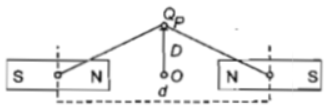The force on the charge Q is

1. zero

2. directed along OP

3. directed along PO

4. directed perpendicular to the plane of the paper

Concept Questions :-

Bar magnet